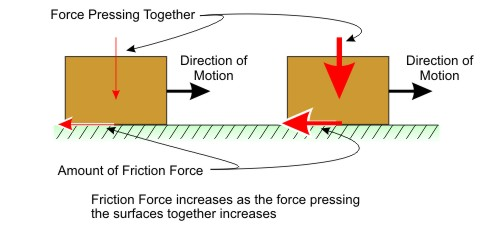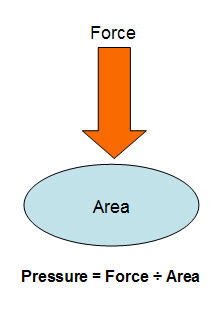## Friday, April 25, 2014

### 8th Force And Pressure "Notes and Questions Answers"

A force is an agent which can change or try to change the state of an object. Force is the product of  mass and acceleration
F = ma
Force has both magnitude and direction, making it a vector quantity. The SI unit used to measure force is the Newton (symbol N), which is equivalent to kg•m•s-2

Actions like picking, opening, shutting, kicking, hitting, lifting, flicking, pushing, pulling are often used to describe certain tasks. Each of these actions usually results in some kind of change in the motion of an object.
A force is a push or pull upon an object resulting from the object's interaction with another object.

Whenever there is an interaction between two objects, there is a force upon each of the objects. When the interaction ceases, the two objects no longer experience the force. Forces only exist as a result of an interaction.

All forces (interactions) between objects can be placed into two broad categories:

• Contact forces, and
• Non Contact forces

Contact Forces: Contact forces are those types of forces which result when the two interacting objects are perceived to be physically contacting each other. Examples of contact forces include frictional forces, tensional forces, normal forces, air resistance forces, and applied forces.

Non-contact Forces: Non Contact forces are those types of forces which result even when the two interacting objects are not in physical contact with each other, yet are able to exert a push or pull despite their physical separation. Examples of Non Contact forces include gravitational forces.

Types of Contact Forces

Applied Force: - An applied force is a force, which is applied to an object by a person or another object. For example, If a person is pushing a desk across the room, then there is applied force acting upon the object. The applied force is the force exerted on the desk by the person.

Normal Force: - The normal force is the support force exerted upon an object which is in contact with another stable object. For example, if a book is resting upon a surface, then the surface is exerting an upward force upon the book in order to support the weight of the book. On occasions, a normal force is exerted horizontally between two objects which are in contact with each other. For instance, if a person leans against a wall, the wall pushes horizontally on the person.

Frictional Force: - The friction force is the force exerted by a surface as an object moves across it or makes an effort to move across it. There are at least two types of friction force - sliding and static friction. For example, if a book slides across the surface of a desk, then the desk exerts a friction force in the opposite direction of its motion. Friction results from the two surfaces being pressed together closely, causing intermolecular attractive forces between molecules of different surfaces. As such, friction depends upon the nature of the two surfaces and upon the degree to which they are pressed together.

Friction is necessary for every movement in life. Suppose you spill some oil on the floor then it will be difficult for you to walk because of negligible friction.Air Resistance Force: - The air resistance is a special type of frictional force which acts upon objects as they travel through the air. The force of air resistance is often observed to oppose the motion of an object. This force will frequently be neglected due to its negligible magnitude (and due to the fact that it is mathematically difficult to predict its value). It is most noticeable for objects which travel at high speeds (e.g., a skydiver or a downhill skier) or for objects with large surface areas. When you ride your bike then your hair flip backwards because of air resistance.

Tension Force: - The tension force is the force which is transmitted through a string, rope, cable or wire when it is pulled tight by forces acting from opposite ends. The tension force is directed along the length of the wire and pulls equally on the objects on the opposite ends of the wire.

Spring Force: - The spring force is the force exerted by a compressed or stretched spring upon any object which is attached to it. An object which compresses or stretches a spring is always acted upon by a force which restores the object to its rest or equilibrium position.

For most springs (specifically, for those which are said to obey "Hooke's Law"), the magnitude of the force is directly proportional to the amount of stretch or compression of the spring.

Types of Non-contact Forces

Gravitational Force: - The force of gravity is the force with which the earth, moon, or other massively large object attracts another object towards itself. By definition, this is the weight of the object. All objects upon earth experience a force of gravity which is directed "downward" towards the center of the earth. The force of gravity on earth is always equal to the weight of the object as found by the equation:
F grav = m x g
where g = 9.8 m/s2 (on Earth) and m = mass (in kg)

Magnetic Force:- Attraction or repulsion that arises between electrically charged particles because of their motion; the basic force responsible for the action of electric motors and the attraction of magnets for iron. Electric forces exist among stationary electric charges; both electric and magnetic forces exist among moving electric charges. The magnetic force between two moving charges may be described as the effect exerted upon either charge by a magnetic field created by the other.

The magnetic force on a moving charge is exerted in a direction at a right angle to the plane formed by the direction of its velocity and the direction of the surrounding magnetic field.

Electrostatic Force: - The force exerted by stationary objects bearing electric charge on other stationary objects bearing electric charge. If the charges are of the same sign, then the force is repulsive; if they are of opposite signs, the force is attractive. The strength of the force is described by Coulomb's law. Also called electrostatic force.

The force exerted by a charged body on another charged or uncharged body is known as electrostatic force. This force comes into play even when the bodies are not in contact.

Try making your friend sit on a plastic chair with feet not touching the ground. Then use a towel to rub on the chair or on your friend. After about 30 second of continuing this activity, touch your friend. You will get a mild electric shock. This is because of electrostatic charge in the chair and in your friend’s body. You can notice a layer of dust always sticking to TV screen. This happens because of electrostatic charge.

Pressure

Pressure (symbol: p or sometimes P) is the force per unit area applied to an object in a direction perpendicular to the surface.

Mathematically:

P = F/A

Where: p is the pressure,  F is the normal force,  A is the area

The SI unit for pressure is the pascal (Pa), equal to one newton per square meter (N•m-2 or kg•m-1•s-2).Friction:  Friction is the force resisting the relative lateral (tangential) motion of solid surfaces, fluid layers, or material elements in contact.

Force of Friction :

Friction is a force that is created whenever two surfaces move or try to move across each other.
• Friction always opposes the motion or attempted motion of one surface across another surface.
• Friction is dependant on the texture of both surfaces.
• Friction is also dependant on the amount of contact force pushing the two surfaces together (normal force).

Factors affecting Friction

Friction depends partly on the smoothness of the contacting surfaces, a greater force being needed to move two surfaces past one another if they are rough than if they are smooth. However, friction decreases with smoothness only to a degree; friction actually increases between two extremely smooth surfaces because of increased attractive electrostatic forces between their atoms.

Friction does not depend on the amount of surface area in contact between the moving bodies or (within certain limits) on the relative speed of the bodies. It does, however, depend on the magnitude of the forces holding the bodies together.
When a body is moving over a horizontal surface, it presses down against the surface with a force equal to its weight, i.e., to the pull of gravity upon it; an increase in the weight of the body causes an increase in the amount of resistance offered to the relative motion of the surfaces in contact.

Types of Friction

Static friction: - Static friction is friction between two solid objects that are not moving relative to each other. For example, static friction can prevent an object from sliding down a sloped surface. The coefficient of static friction, typically denoted as μs, is usually higher than the coefficient of kinetic friction.
An example of static friction is the force that prevents a car wheel from slipping as it rolls on the ground. Even though the wheel is in motion, the patch of the tire in contact with the ground is stationary relative to the ground, so it is static rather than kinetic friction.

The maximum value of static friction, when motion is impending, is sometimes referred to as limiting friction, although this term is not used universally.

Kinetic friction:- Kinetic (or dynamic) friction occurs when two objects are moving relative to each other and rub together (like a sled on the ground). The coefficient of kinetic friction is typically denoted as μk, and is usually less than the coefficient of static friction for the same materials.

• Kinetic friction is when two objects are rubbing against each other. Putting a book flat on a desk and moving it around is an example of kinetic friction.

• Fluid friction is the interaction between a solid object and a fluid (liquid or gas), as the object moves through the fluid. The skin friction of air on an airplane or of water on a swimmer are two examples of fluid friction.

Reducing friction :

Devices: Devices such as wheels, ball bearings, air cushion or roller bearing can change sliding friction into a much smaller type of rolling friction. Many thermoplastic materials such as nylon, HDPE and PTFE are commonly used for low friction bearings. They are especially useful because the coefficient of friction falls with increasing imposed load.

Lubricants: A common way to reduce friction is by using a lubricant, such as oil, water, or grease, which is placed between the two surfaces, often dramatically lessening the coefficient of friction. The science of friction and lubrication is called tribology. Lubricant technology is when lubricants are mixed with the application of science, especially to industrial or commercial objectives.

Energy of friction

According to the law of conservation of energy, no energy is destroyed due to friction, though it may be lost to the system of concern. Energy is transformed from other forms into heat.

 1.Force ,Frictions and Pressure CBSE Practice Assignment Key concept for Quick revision Worksheet  based on “ Calculate Net force” Force , friction and pressure Study Notes solve Questions [Summative  Assessment]
More related search:
MCQ:Thrust , Presure,Buyoancy and Density